Next: Sinusoidal waveshaping: evenness and Up: Examples Previous: Waveshaping using Chebychev polynomials   Contents   Index

## Waveshaping using an exponential function

We return again to the spectra computed on Page, corresponding to waveshaping functions of the form. We note with pleasure that not only are they all in phase (so that they can be superposed with easily predictable results) but also that the spectra spread out asincreases. Also, in a series of the form,a higher index of modulation will lend more relative weight to the higher power terms in the expansion; as we saw seen earlier, if the index of modulation is, the various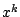terms are multiplied by,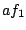,, and so on.

Now suppose we wish to arrange for different terms in the above expansion to dominate the result in a predictable way as a function of the index. To choose the simplest possible example, suppose we wishto be the largest term for, then for it to be overtaken by the more quickly growingterm for, which is then overtaken by theterm for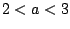and so on, so that each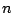th term takes over at index. To make this happen we just require thatand so choosing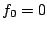, we get,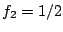,, and in general,These happen to be the coefficients of the power series for the function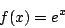whereis Euler's constant.

Before plugging inas a transfer function it's wise to plan how we will deal with signal amplitude, sincegrows quickly as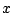increases. If we're going to plug in a sinusoid of amplitude, the maximum output will be, occurring whenever the phase is zero. A simple and natural choice is simply to divide byto reduce the peak to one, giving:This is realized in Example E06.exponential.pd. Resulting spectra for0, 4, and 16 are shown in Figure 5.13. As the waveshaping index rises, progressively less energy is present in the fundamental; the energy is increasingly spread over the partials.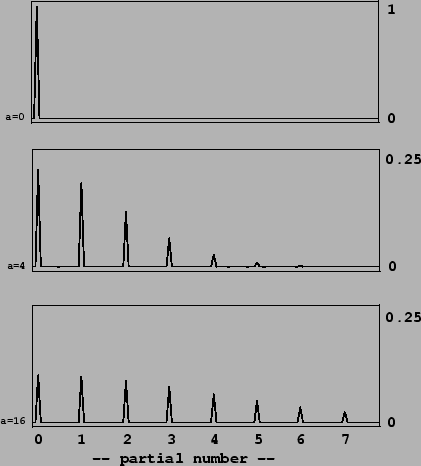Next: Sinusoidal waveshaping: evenness and Up: Examples Previous: Waveshaping using Chebychev polynomials   Contents   Index
Miller Puckette 2006-12-30